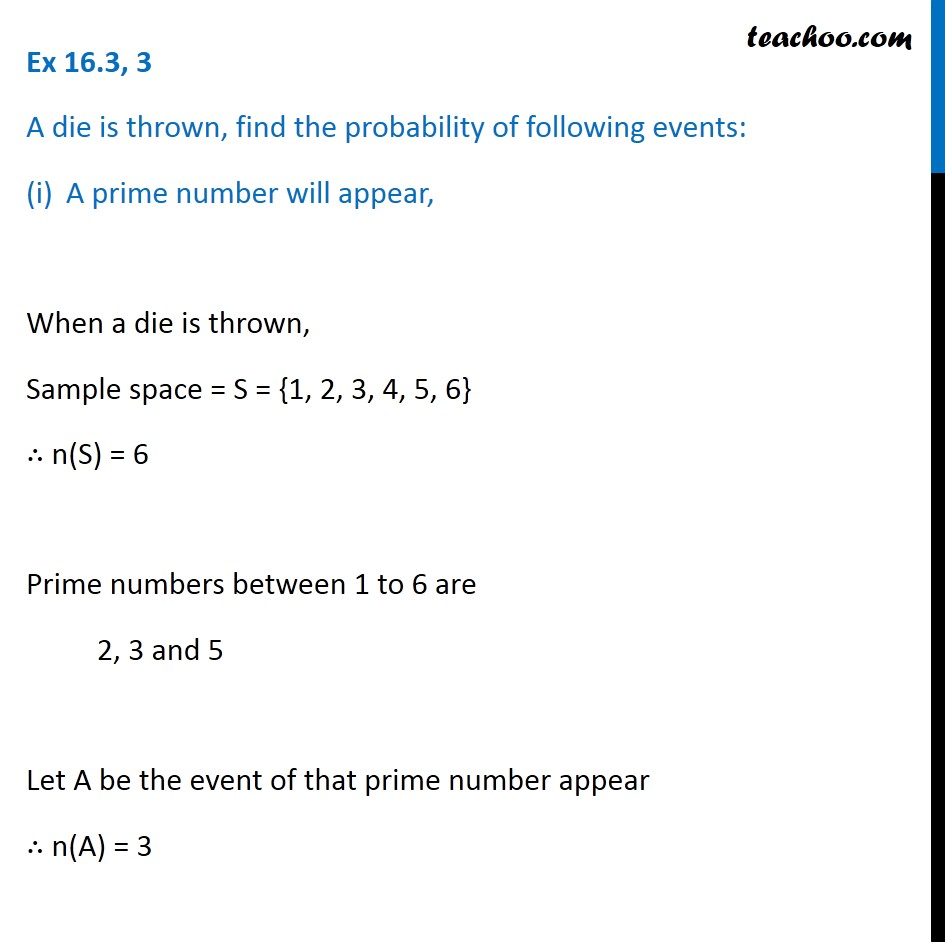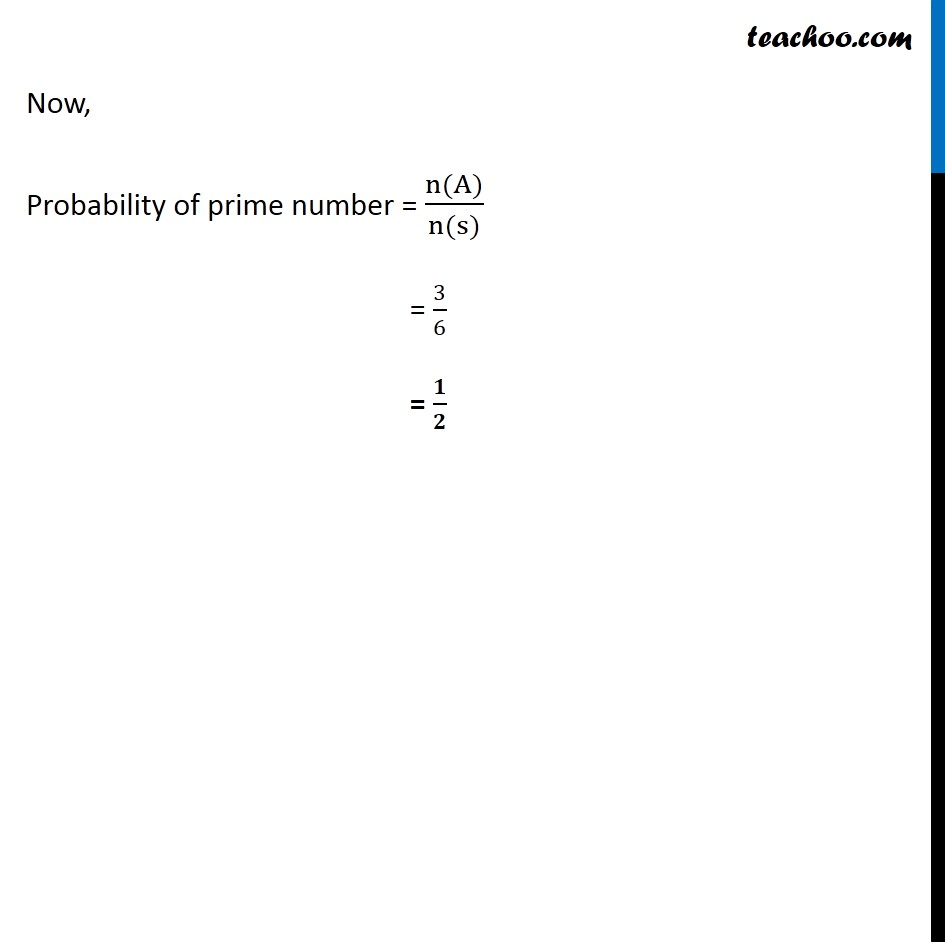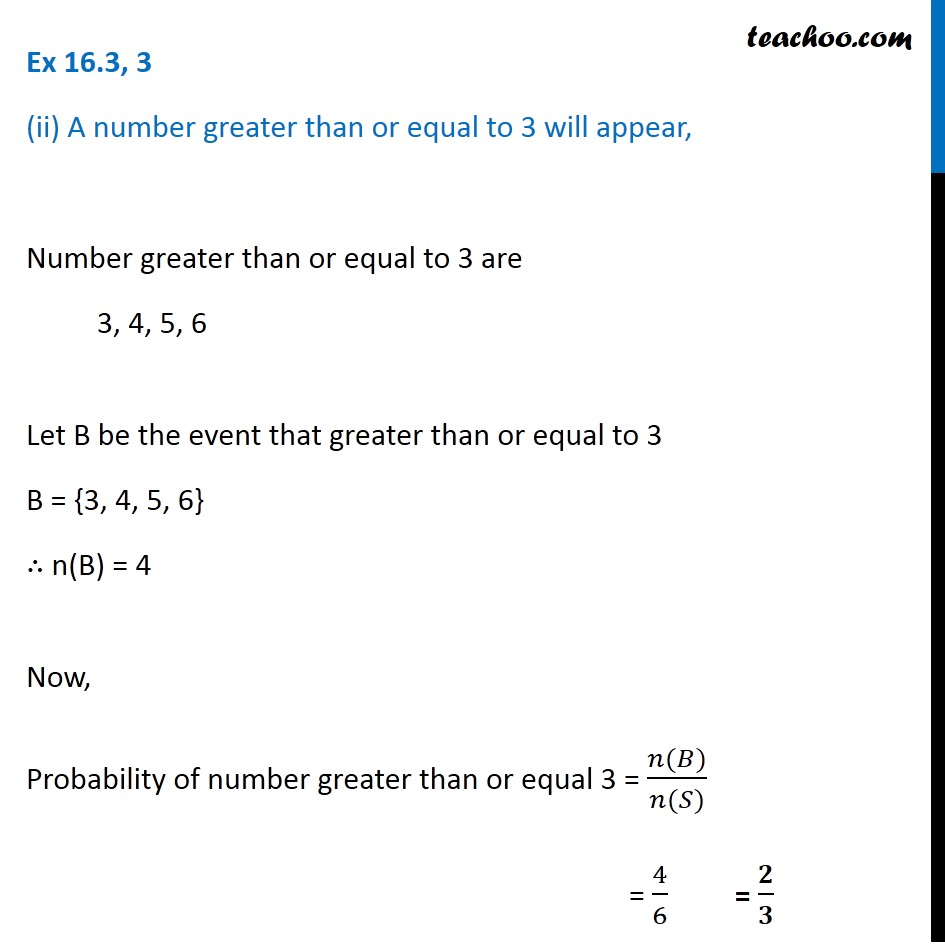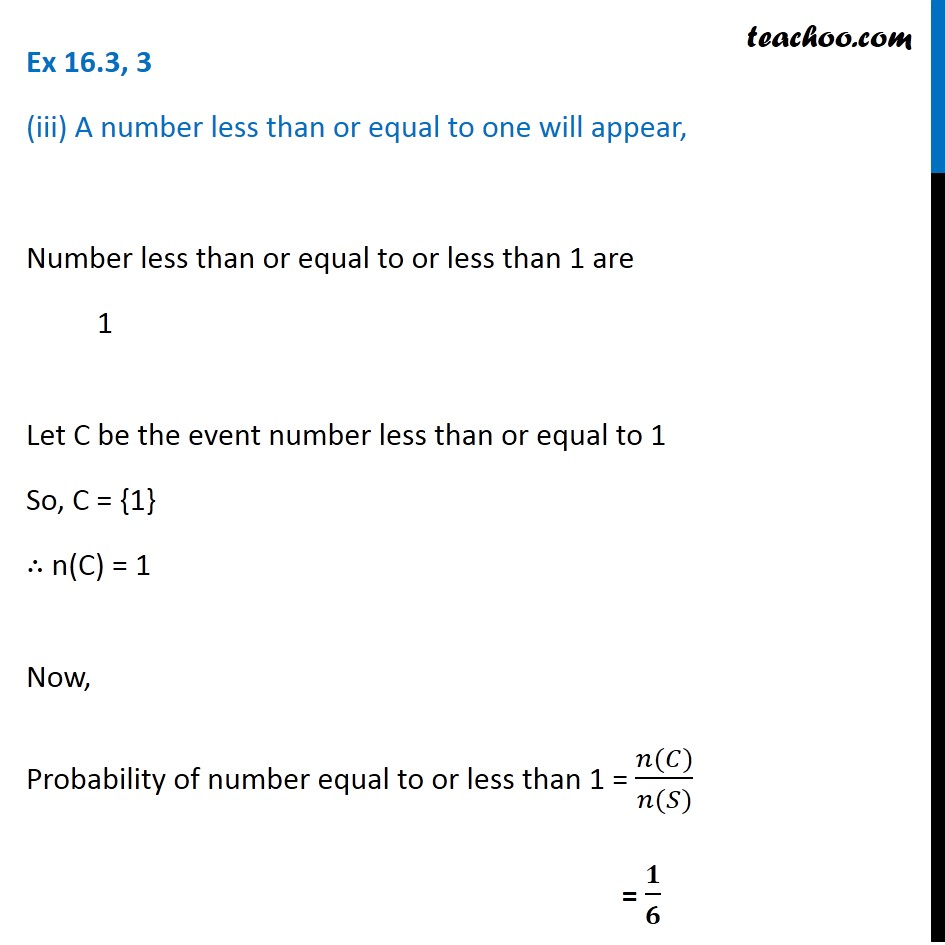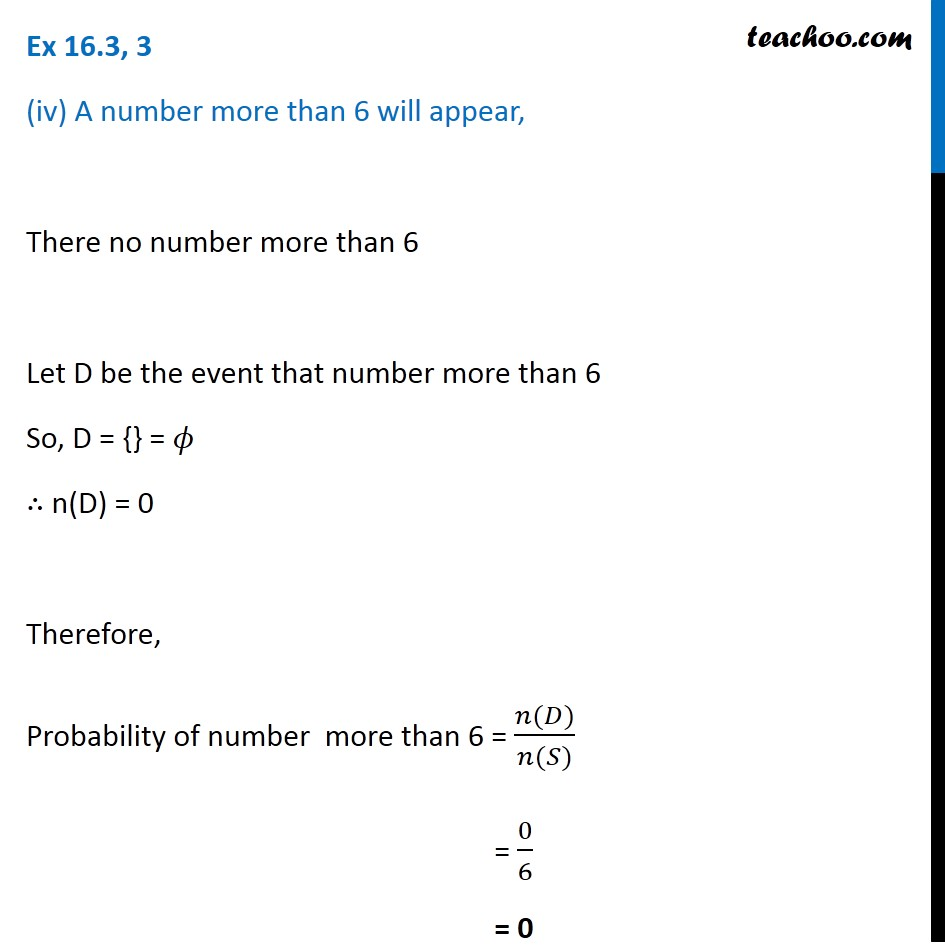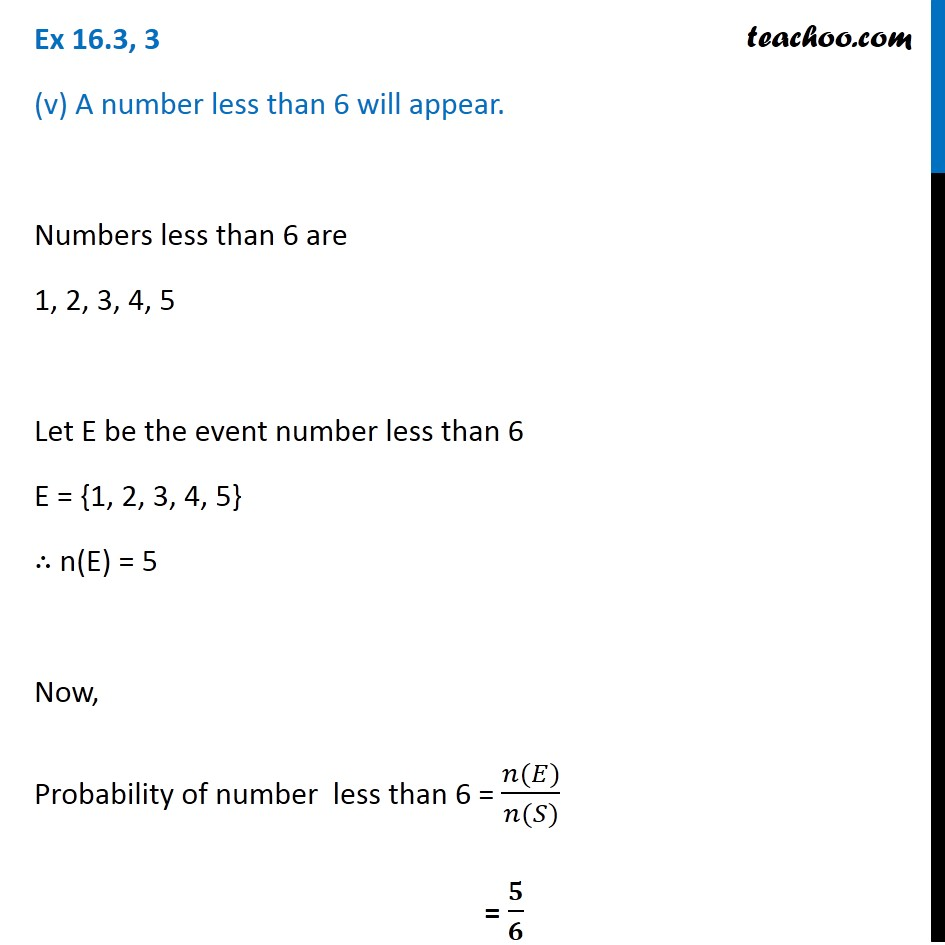Subscribe to our Youtube Channel - https://you.tube/teachoo

1. Chapter 16 Class 11 Probability
2. Serial order wise
3. Ex 16.3

Transcript

Ex 16.3, 3 A die is thrown, find the probability of following events: A prime number will appear, When a die is thrown, Sample space = S = {1, 2, 3, 4, 5, 6} ∴ n(S) = 6 Prime numbers between 1 to 6 are 2, 3 and 5 Let A be the event of that prime number appear ∴ n(A) = 3 Now, Probability of prime number = (n(A))/(n(s)) = 3/6 = 𝟏/𝟐 Ex 16.3, 3 (ii) A number greater than or equal to 3 will appear, Number greater than or equal to 3 are 3, 4, 5, 6 Let B be the event that greater than or equal to 3 B = {3, 4, 5, 6} ∴ n(B) = 4 Now, Probability of number greater than or equal 3 = (𝑛(𝐵))/(𝑛(𝑆)) = 4/6 = 𝟐/𝟑 Ex 16.3, 3 (iii) A number less than or equal to one will appear, Number less than or equal to or less than 1 are 1 Let C be the event number less than or equal to 1 So, C = {1} ∴ n(C) = 1 Now, Probability of number equal to or less than 1 = (𝑛(𝐶))/(𝑛(𝑆)) = 𝟏/𝟔 Ex 16.3, 3 (iv) A number more than 6 will appear, There no number more than 6 Let D be the event that number more than 6 So, D = {} = 𝜙 ∴ n(D) = 0 Therefore, Probability of number more than 6 = (𝑛(𝐷))/(𝑛(𝑆)) = 0/6 = 0 Ex 16.3, 3 (v) A number less than 6 will appear. Numbers less than 6 are 1, 2, 3, 4, 5 Let E be the event number less than 6 E = {1, 2, 3, 4, 5} ∴ n(E) = 5 Now, Probability of number less than 6 = (𝑛(𝐸))/(𝑛(𝑆)) = 𝟓/𝟔

Ex 16.3